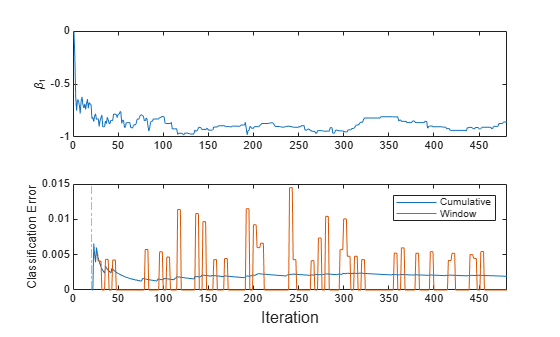Implement Incremental Learning for Classification Using Succinct Workflow

This example shows how to use the succinct workflow to implement incremental learning for binary classification with prequential evaluation. Specifically, this example does the following:

1. Create a default incremental learning model for binary classification.

2. Simulate a data stream using a for loop, which feeds small chunks of observations to the incremental learning algorithm.

3. For each chunk, use updateMetricsAndFit to measure the model performance given the incoming data, and then fit the model to that data.

Although this example treats the application as a binary classification problem, you can implement multiclass incremental learning using the naive Bayes algorithm instead by following this same workflow. See the incrementalClassificationNaiveBayes object.

Create Default Model Object

Create a default incremental learning model for binary classification.

Mdl = incrementalClassificationLinear()
Mdl =
incrementalClassificationLinear

IsWarm: 0
Metrics: [1x2 table]
ClassNames: [1x0 double]
ScoreTransform: 'none'
Beta: [0x1 double]
Bias: 0
Learner: 'svm'

Properties, Methods

Mdl is an incrementalClassificationLinear model object. All its properties are read-only.

Mdl must be fit to data before you can use it to perform any other operations.

Load the human activity data set. Randomly shuffle the data.

n = numel(actid);
rng(1); % For reproducibility
idx = randsample(n,n);
X = feat(idx,:);
Y = actid(idx);

For details on the data set, enter Description at the command line.

Responses can be one of five classes: Sitting, Standing, Walking, Running, or Dancing. Dichotomize the response by identifying whether the subject is moving (actid > 2).

Y = Y > 2;

Implement Incremental Learning

Use the succinct workflow to update model performance metrics and fit the incremental model to the training data by calling the updateMetricsAndFit function. At each iteration:

• Process 50 observations to simulate a data stream.

• Overwrite the previous incremental model with a new one fitted to the incoming observation.

• Store the cumulative metrics, the window metrics, and the first coefficient ${\beta }_{1}$ to see how they evolve during incremental learning.

% Preallocation
numObsPerChunk = 50;
nchunk = floor(n/numObsPerChunk);
ce = array2table(zeros(nchunk,2),'VariableNames',["Cumulative" "Window"]);
beta1 = zeros(nchunk,1);

% Incremental fitting
for j = 1:nchunk
ibegin = min(n,numObsPerChunk*(j-1) + 1);
iend   = min(n,numObsPerChunk*j);
idx = ibegin:iend;
Mdl = updateMetricsAndFit(Mdl,X(idx,:),Y(idx));
ce{j,:} = Mdl.Metrics{"ClassificationError",:};
beta1(j + 1) = Mdl.Beta(1);
end

IncrementalMdl is an incrementalClassificationLinear model object trained on all the data in the stream. During incremental learning and after the model is warmed up, updateMetricsAndFit checks the performance of the model on the incoming observation, and then fits the model to that observation.

Inspect Model Evolution

To see how the performance metrics and ${\beta }_{1}$ evolved during training, plot them on separate subplots.

figure;
subplot(2,1,1)
plot(beta1)
ylabel('\beta_1')
xlim([0 nchunk]);
xline(Mdl.EstimationPeriod/numObsPerChunk,'r-.');
subplot(2,1,2)
h = plot(ce.Variables);
xlim([0 nchunk]);
ylabel('Classification Error')
xline(Mdl.EstimationPeriod/numObsPerChunk,'r-.');
xline((Mdl.EstimationPeriod + Mdl.MetricsWarmupPeriod)/numObsPerChunk,'g-.');
legend(h,ce.Properties.VariableNames)
xlabel('Iteration')The plot suggests that updateMetricsAndFit does the following:

• Fit ${\beta }_{1}$ during all incremental learning iterations.

• Compute performance metrics after the metrics warm-up period only.

• Compute the cumulative metrics during each iteration.

• Compute the window metrics after processing 200 observations (4 iterations).